# High School Math : High School Math

## Example Questions

### Example Question #51 : High School Math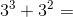Possible Answers: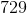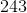Correct answer:Explanation:

Even though the base of the exponents are the same, you cannot add the exponents. You must perform each exponent separately.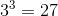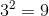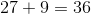### Example Question #52 : High School Math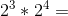Possible Answers: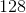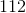Correct answer:Explanation:

Since the two bases of the exponents are the same and are being multiplied, it is acceptable to combine the terms and add their exponents resulting in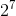which equals 128.

### Example Question #1 : Integers And Types Of Numbers

Isan integer, decimal, or a fraction?

Possible Answers:

Decimal

None

Integer

Fraction

Correct answer:

Fraction

Explanation:

A fraction is a number with a numerator and denominator that can not be simplified into an integer so the numberis a fraction.

### Example Question #1 : Integers And Types Of Numbers

Which of the following is an integer?

Possible Answers: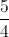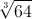1.5

-100.1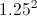Correct answer:Explanation:

Integers are any number that doesn't have anything in the decimal places.  They are also known as whole numbers.  The cubed root of 64 is 4, which is the only whole number out of the answer choices.

### Example Question #1 : Integers And Types Of Numbers

Which of the following is NOT an integer?

Possible Answers: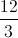-4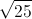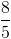1024

Correct answer:Explanation:

An integer is simply a whole negative or positive number, which means it can be expressed without decimal places.  Any number can be considered an integer as long as it fits this criteria.  They can be expressed as square roots, fractions, or anything else, as long as the final answer is a whole number.  In this question, the only answer that is not a whole number is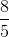, which is 1.6.

### Example Question #51 : High School Math

Which of the following describes the number?

Possible Answers:

Rational

All of these

Integer

Real

Correct answer:

All of these

Explanation:

A real number is anything that is not imaginary, including all rational and irrational numbers.

A rational number is any number that can be expressed as a fraction of two integers.

An integer is any whole number, including zero.is not imaginary, can be expressed as a fraction, and is a whole number; thus, it falls into all of these categories.

### Example Question #52 : High School Math

Find the greatest common factor and the least common multiple ofand.  What is the sum of the GCF and LCM?

Possible Answers: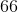Correct answer:Explanation:

Prime factorize each number.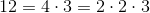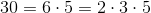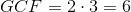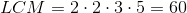Thus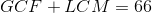.

### Example Question #11 : Number Theory

Which of the following is not an integer?

Possible Answers: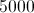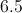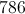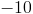Correct answer:Explanation:

By definition, an integer is a whole number that is either positive or negative. Negative numbers such as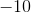andare integers, as are 10 and 5.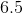, however, is not an integer because it isn't a whole number.

### Example Question #11 : Integers And Types Of Numbers

What type of number is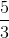?

Possible Answers:

Irrational

Fraction

Integer

Decimal

None

Correct answer:

Fraction

Explanation:

An integer is a whole number, either positive or negative. In other words, it is a number that can be written without a fractional or decimal part. Examples are: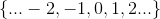is therefore not an integer, but it is a fraction.

### Example Question #21 : Number Theory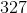is an example of _____________.

Possible Answers:

an irrational number

a fraction

a complex number

a decimal

an integer

Correct answer:

an integer

Explanation:

An integer is any positive or negative whole number.

Therefore,is an integer.

### All High School Math Resources